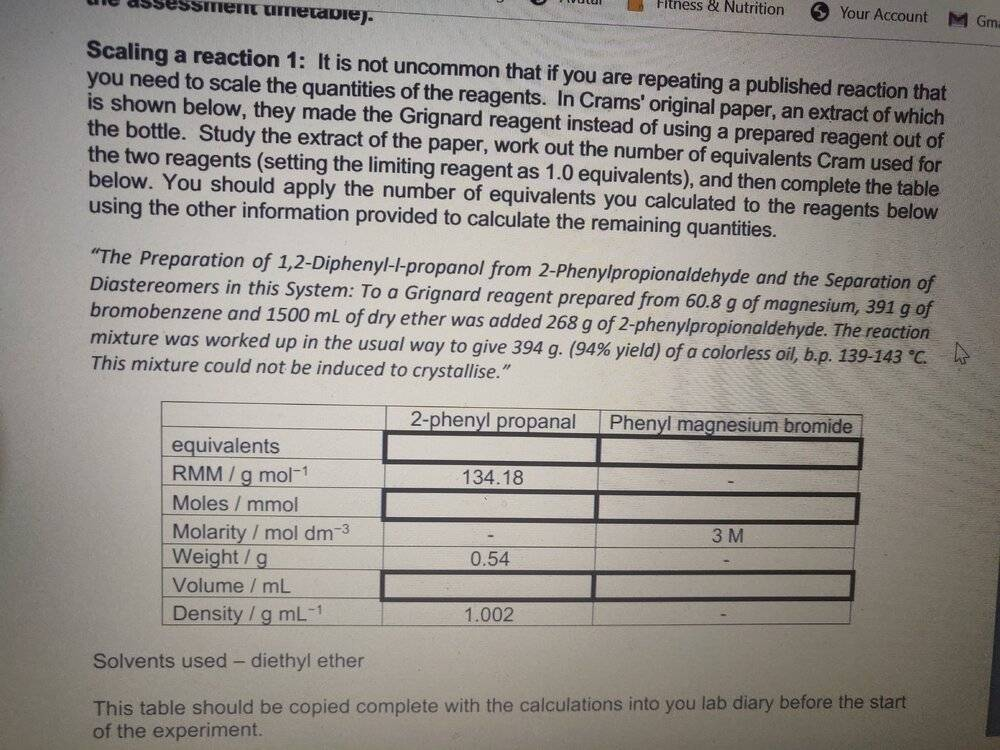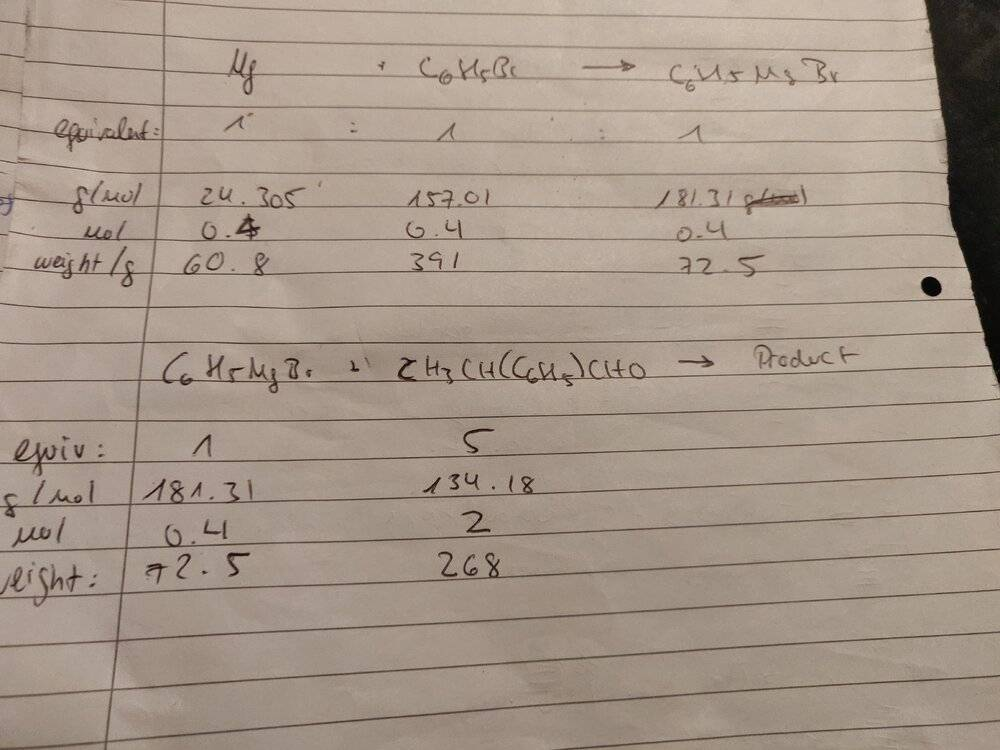# Help with mol and volume calculations - Cram's rule

• Chemistry
Yokoko
Homework Statement:
I've been struggling with this table as the description makes little sense to me.

I'm a 2nd year chemistry student who has changed courses this year so I have never done chemistry this intensively before.
Relevant Equations:
/Am I right to assume that the equivalents for phenyl magnesium bromide would be 3 (magnesium, bromobenzene and ether (?)) And the number for 2-phenyl-propanal 1 as it says "...was added to 2-phenylpropionaldehyde" which is the same thing as 2-phenylpropanal? I don't know if I understood this correctly.

Also irregardless, would my calculations then be right e.g. for the moles of 2-phenyl propanal being 0.00371

Using the formula m/M=n (0.54/134.18)?

Thanks guys!

Mentor
Am I right to assume that the equivalents for phenyl magnesium bromide would be 3 (magnesium, bromobenzene and ether (?))

No. You need to find out how much phenyl magnesium bromide can be prepared from the given amounts of magnesium and phenyl bromide. And ether is just a solvent.

added to 2-phenylpropionaldehyde" which is the same thing as 2-phenylpropanal?

Yes, it is the same thing. A bit lousy naming but perfectly unambiguous.

Also irregardless, would my calculations then be right e.g. for the moles of 2-phenyl propanal being 0.00371

Using the formula m/M=n (0.54/134.18)?

No.

Actually I have no idea what 0.54 is and where did it came from.

Start by finding out how many moles of phenyl magnesium bromide were produced. What is the stoichiometric amount of the aldehyde that should react with it? What is the amount used?

Yokoko
I have worked the following out: 72.5g of phenyl magnesium bromide are prepared from the reagents.

I tried to take it a step further and create the same table for the reaction of phenyl magnesium bromide and 2-phenyl propanal but I'm unsure whether I can infer it like that - especially given that the weight values are different from the exercise...The 0.54 was the weight in g of 2 phenyl propanal as indicated in the table below the exercise description.

Mentor
I have worked the following out: 72.5g of phenyl magnesium bromide are prepared from the reagents.

No.

Sanity check: you have 60.8 g of Mg, molar mass of magnesium is 24.3 g/mol, is it possible to have 0.4 moles of Mg if you have several times more than the molar mass?

It is a bit like saying "I have 60 marbles, box holds 24, so it will be filled in 40%".

I tried to take it a step further and create the same table for the reaction of phenyl magnesium bromide and 2-phenyl propanal

That's what you will have to do, but you are doing some strange things - what does "5 equivalents" mean and where does this number come from?

The 0.54 was the weight in g of 2 phenyl propanal as indicated in the table below the exercise description.

Can't be, you are told there were 268 g of the aldehyde used.

On the second thought: that's probably mass of the aldehyde you are expected to do calculations for, using the data from the Cram's paper (as if you were planning to do the reaction using 0.54 g of the aldehyde instead of 268 g used by Cram).

Yokoko
Apologies, that makes sense! It seems like I put the equation the wrong way around ie calculated 24.305/60.8 instead of 60.8/24.305.

So the moles for each of the compounds are 2.5, leaving me with 453.28g of phenyl magnesium bromide

The equivalent numbers were probably this wrong because I induced it from the miscalculated moles from earlier. Using 2.5 moles for phenyl magnesium bromide, the ratio in the second table should be 1:1.25

Does that mean the equivalent would also be 1:1.25 in the original table, after which the exercise requires me to calculate the remaining values assuming I only use 0.54g of the aldehyde?